##Mathematics Field Days For New England High School Students Answers to the Fractal Quiz!!!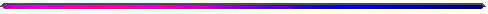1A. Here is a target for the chaos game. Using a sequence of A's, B's, and C's, explain how to move the seed into the interior of the target in exactly 5 moves. For example, ABCCC means first do A, then do B, then do CCC.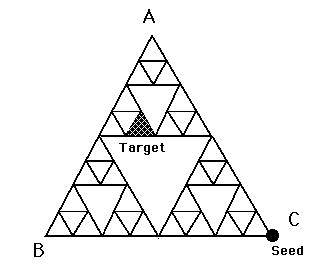Solution: BACBA or ABCBA

1B. Here is a second target.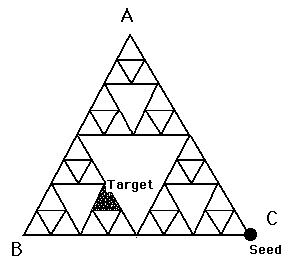Solution: BAACB or ABACB

2. Explain how we generated each of the following fractal images. Specifically, you need to provide

• The number of vertices
• The compression ratio(s)
• The rotations (if any)

A.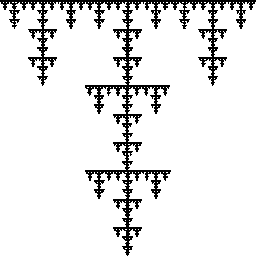Solution:

Number of vertices = 5
Compression ratio = 3
Rotations = none

B.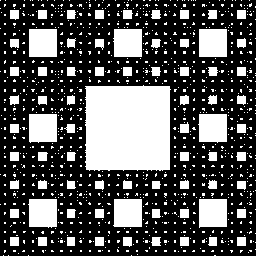Solution:

Number of vertices = 8
Compression ratio = 3
Rotations = none

C.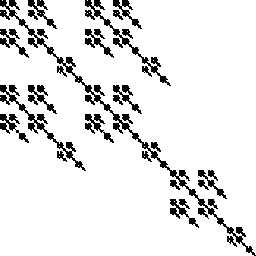Solution:

Number of vertices = 5
Compression ratio = 3
Rotations = none

D.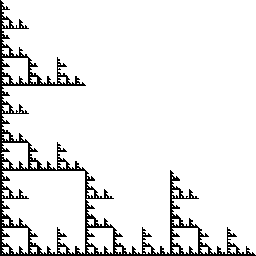Solution:

Number of vertices = 5
Compression ratio = 3
Rotations = none

E.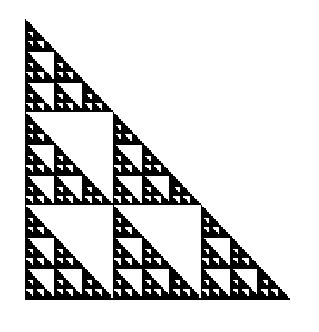Solution:

Number of vertices = 6
Compression ratio = 3
Rotations = none

F.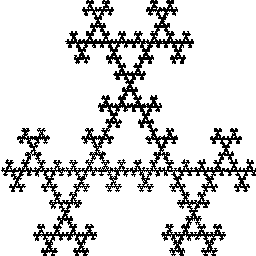Solution:

Number of vertices = 3
Compression ratio = 2
Rotations = 180

 3. How did we make this animation? How many vertices in each frame? What is the compression ratio? In a brief paragraph, explain how the motion changes from frame to frame.Solution:

Number of vertices = 3
Compression ratio = 2
The topmost vertex was rotated 30 degrees at each different frame of the film.

 4. Now how did we make this animation? How many vertices in each frame? What is the compression ratio? In a brief paragraph, explain how the motion changes from frame to frame.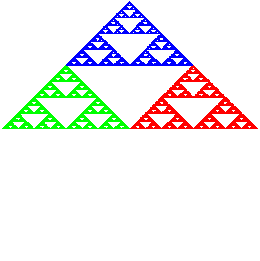Solution:

Number of vertices = 3
Compression ratio = 2
The center vertex oscillated up and down along the vertical axis. Meanwhile the rotations around this vertex increased by 18 degrees frame of the film.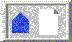BU Dynamical Systems and Technology Project